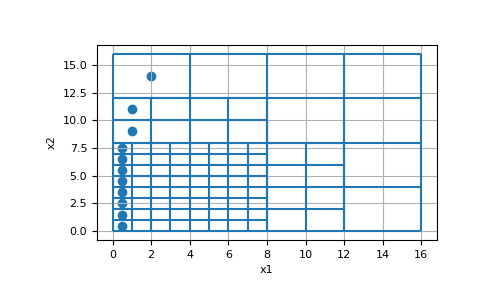# discretize.TreeMesh.cell_boundary_indices¶

TreeMesh.cell_boundary_indices

Returns the indices of the x, y (and z) boundary cells

This property returns the indices of the cells on the x, y (and z) boundaries, respectively. Note that each axis direction will have both a lower and upper boundary. The property will return the indices corresponding to the lower and upper boundaries separately.

E.g. for a 2D domain, there are 2 x-boundaries and 2 y-boundaries (4 in total). In this case, the return is a list of length 4 organized [ind_Bx1, ind_Bx2, ind_By1, ind_By2]:

```           By2
+ ------------- +
|               |
|               |
Bx1 |               | Bx2
|               |
|               |
+ ------------- +
By1
```
Returns
ind_bx1 ind_bx2, ind_by1, ind_by2`numpy.ndarray` `of` `int`

The length of each array in the list is equal to the number of faces on that particular boundary.

ind_bz1, ind_bz2`numpy.ndarray` `of` `int`, `optional`

Returned if dim is 3.

Examples

Here, we construct a small 2D tree mesh and return the indices of the x and y-boundary cells.

```>>> from discretize import TreeMesh
>>> from discretize.utils import refine_tree_xyz
>>> import numpy as np
>>> import matplotlib.pyplot as plt
```
```>>> hx = np.ones(16)
>>> hy = np.ones(16)
>>> mesh = TreeMesh([hx, hy])
>>> mesh.refine_ball([4.0,4.0], [4.0], )
>>> ind_Bx1, ind_Bx2, ind_By1, ind_By2 = mesh.cell_boundary_indices
```
```>>> ax = plt.subplot(111)
>>> mesh.plot_grid(ax=ax)
>>> ax.scatter(*mesh.cell_centers[ind_Bx1].T)
>>> plt.show()
```